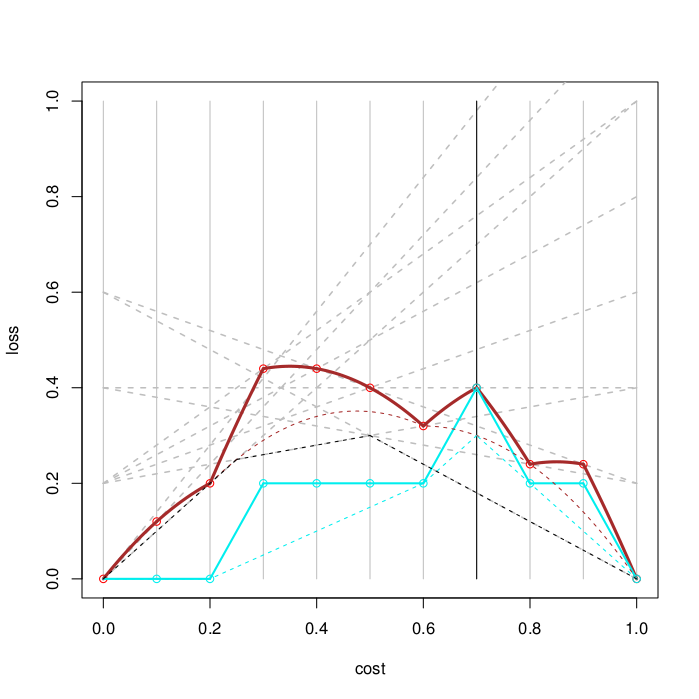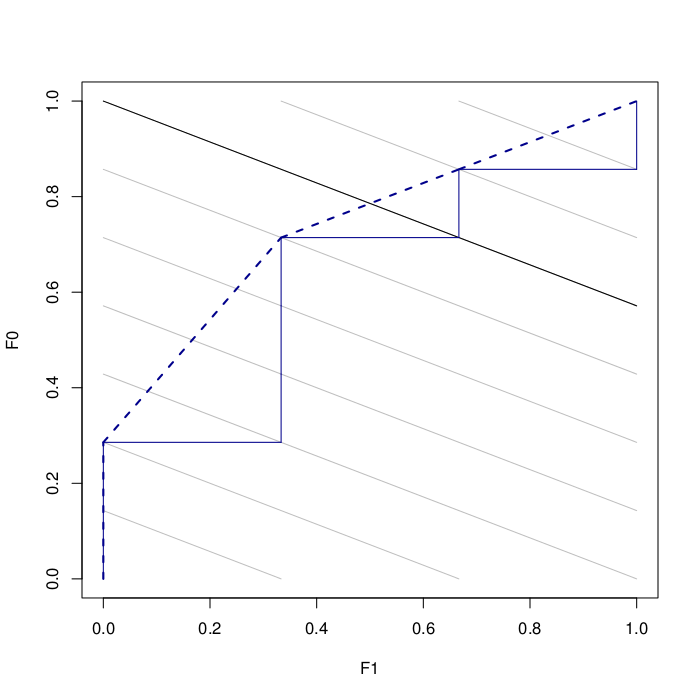# Rate Driven Curves: ROC Curves in Cost Space•  ROC curves can be transferred to cost space by means of a very natural threshold choice method:
• We employ a very natural threshold choice method, which sets the decision threshold such that the proportion of positive predictions
equals the operating condition
• Using this threshold choice method we define a new curve: rate-driven curves
• The expected loss as measured by the area under these curves is linearly related to AUC
• Rate-driven curves are the genuine equivalent of ROC curves in cost space
• A decomposition of the rate-driven curves separates the loss due to the threshold choice method from the ranking loss (Kendall τ distance)
• The corresponding curve to the ROC convex hull in cost space is also derived

• More details in this paper.
• An R script for drawing Rate-Driven curves and ROC curves.